# RRB PO Prelims Quantitative Aptitude Quiz – 10

## RRB PO Prelims Quantitative Aptitude Quiz

Quantitative Aptitude is the most significant part of almost all competitive exams. Many candidates find difficulty in solving Quantitative Aptitude Questions. The only way to ace this section is to practice as many questions as possible. And, to help you practice more and more questions, we have come up with the RRB PO Quantitative Aptitude Quiz. This RRB PO Quantitative Aptitude Quiz is completely Free. This RRB PO Quantitative Aptitude Quiz includes a variety of questions that are usually asked in preliminary and main exams of various banking exams. Candidates will be provided with a detailed solution for each question in this RRB PO Quantitative Aptitude Quiz. This RRB PO Quantitative Aptitude Quiz will assist candidates in better preparing for upcoming exams.

1. A train takes 3 seconds to cross a pole and 22 seconds to cross a 855 m long bridge. What is the time taken by the train (in seconds) to cross a 215 m long train coming at a speed of 90 km/hr from the opposite direction?
(a) 5
(b) 8
(c) 12
(d) 7
(e) 15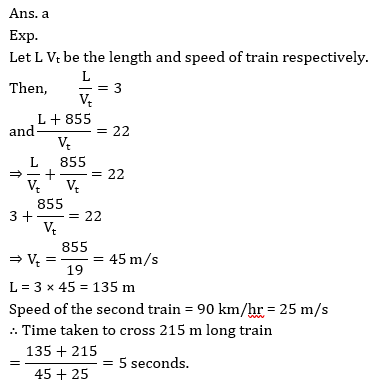2. The numerator of a fraction is increased by 25% and denominator decreased by 75% . The product of new fraction formed and original fraction is 125/16. Find original fraction?
(a) 5 : 3
(b) 5 : 4
(c) 4 : 3
(d) 3 : 5
(e) 4 : 7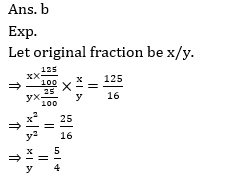3. A, B and C started a business by investing in the ratio 3 : 4 : 5. After 3 months, A withdrew 1/12th of total amount invested by B and C together. If B got Rs. 19200 at end of year as profit, Find share of C in profit?
(a) Rs. 28000
(b) Rs. 22000
(c) Rs. 32000
(d) Rs. 24000
(e) Rs. 35000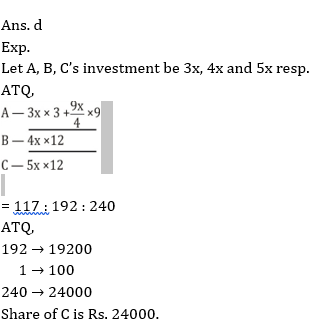4. Average weight of a group is 20 kg. When 2 people include in the group average weight becomes 22 kg after that when 4 another people removed from the group, having weight half of the weight of 2 person included previously, average weight become 25. Find the strength of group initially
(a) 18
(b) 20
(c) 22
(d) 16
(e) 145. Two equal amounts lend on S.I. and C.I. for two years at same rates. If amount after two years is in the ratio of 520 : 529 and difference between interest earned in two years is 18 Rs., then find the ratio of interests earned from S.I to C.I in 3 years if total amount invested in both scheme is 1600 Rs.
(a) 200 : 243
(b) 400 : 463
(c) 200 : 263
(d) 300 : 343
(e) 400 : 443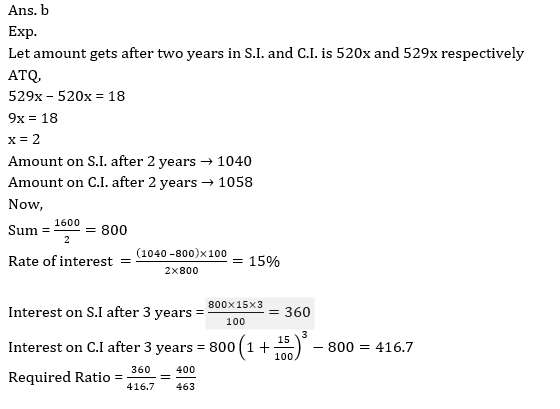Directions (6-10): In each of these questions, two equations (I) and (II) are given. You have to solve both the equations and give answer.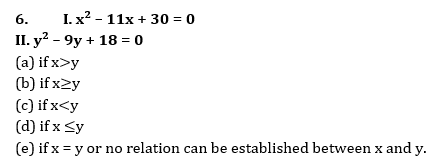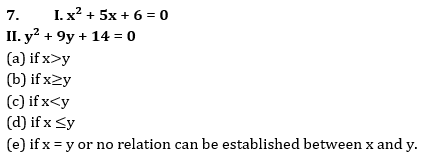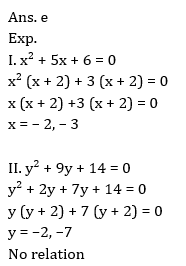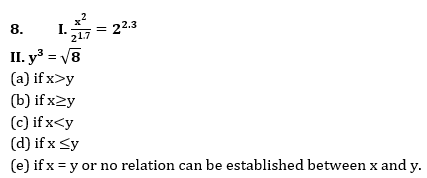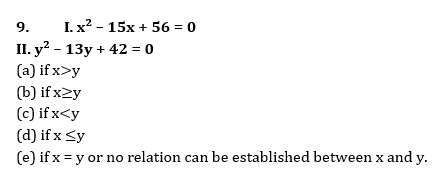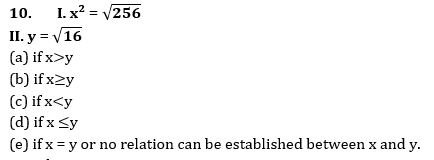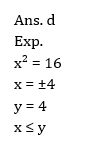###Click to Buy Bank MahaCombo PackageRecommended PDF’s for:

#### Most important PDF’s for Bank, SSC, Railway and Other Government Exam : Download PDF Now

AATMA-NIRBHAR Series- Static GK/Awareness Practice Ebook PDF Get PDF here
The Banking Awareness 500 MCQs E-book| Bilingual (Hindi + English) Get PDF here
AATMA-NIRBHAR Series- Banking Awareness Practice Ebook PDF Get PDF here
Computer Awareness Capsule 2.O Get PDF here
AATMA-NIRBHAR Series Quantitative Aptitude Topic-Wise PDF Get PDF here
AATMA-NIRBHAR Series Reasoning Topic-Wise PDF Get PDF Here
Memory Based Puzzle E-book | 2016-19 Exams Covered Get PDF here
Caselet Data Interpretation 200 Questions Get PDF here
Puzzle & Seating Arrangement E-Book for BANK PO MAINS (Vol-1) Get PDF here
ARITHMETIC DATA INTERPRETATION 2.O E-book Get PDF here
3